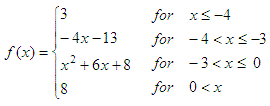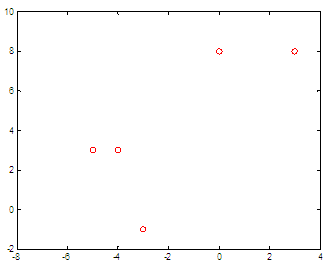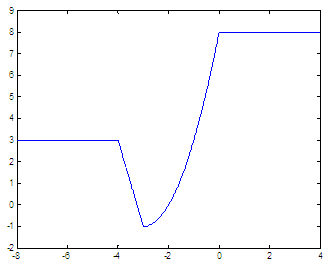# Piecewise function - separate ranges in Matlab

In math, a piecewise function (or piecewise-defined function) is a function whose definition changes depending on the value of the independent variable.

 A function f of a variable x (noted f(x)) is a relationship whose definition is given differently on different subsets of its domain. Piecewise is a term also used to describe any property of a piecewise function that is true for each piece but may not be true for the whole domain of the function. The function doesn’t need to be continuous, it can be defined arbitrarily.

We’re going to experiment in Matlab with this type of functions. We’re going to develop three ways to define and graph them. The first method involves if-statements to classify element-by-element, in a vector. The second method uses switch-case statements, and the third method uses indices to define different sections of the domain.

Let’s say that this is our piecewise-defined functionand we’d like to graph it on the domain of -8 x 4

### Method 1. If-else statements

Notice that this is not the best way to do it in Matlab, but I mention the idea because this is the fundamental concept, and in some programming languages it cannot be done in a dfferent way. It's for study, not for real implementation...

So, let's define our function with if-else statements for the moment. We just use different conditions for the different ranges, and assign appropriate values.

function y = piecewise1(x)
if x <= -4
y = 3;

elseif -4 < x & x <= -3
y = -4*x - 13;

elseif -3 < x & x <= 0
y = x^2 + 6*x + 8;

else
y = 8;
end

Now, we can use scalars or arrays to call this function, in a classical way:

% Define elements
x = [-5 -4 -3 0 3]
% Submit element-by-element to the function
for ix = 1 : length(x)
y(ix) = piecewise1(x(ix))

end
% Plot discrete values
plot(x, y, 'ro')
% Define x and y ranges to display
axis([-8 4 -2 10])

The result is:Let’s say that we need more values. We can call our function this way

x = -8 : .01 : 4;
for ix = 1 : length(x)
y(ix) = piecewise1(x(ix));

end
plot(x, y)
axis([-8 4 -2 9])### Method 2. Switch-case statements

Our second method classifies the elements using switch-case statements. We separate the different ranges in different cases. If that condition is true, then the switch-expression will match the case-expression, and the appropriate statement(s) will be executed.

function  y = piecewise2(x)
switch x

% A scalar switch_expr matches a case_expr if
% switch_expr == case_expr
% The first case must cover the x = 0 case
case x * (-3 < x & x <= 0)
y = x^2 + 6*x + 8;

case x * (x <= -4)
y = 3;

case x * (-4 < x & x <= -3)
y = -4*x - 13;

otherwise
y = 8;
end

One important note is that when x = 0 the result of the case-expression is also 0, and the first case is executed. That’s why we need to place our x = 0 case first in the structure, otherwise we get wrong that point.

We can test our second piecewise function definition, like this:

x = -8 : .01 : 4;
for ix = 1 : length(x)
y(ix) = piecewise2(x(ix));

end
plot(x, y)
axis([-8 4 -2 9])

and get the previous shown graph, too.

### Method 3. Vectorized way

The above routines assume that we’re entering scalars as input parameters. Now, we’ll assume that we’re submitting whole vectors, and we’ll handle indices for that.

This video will show you how to do it without using loops. After the video, another example is given with full code.

The line

x2 = x(-4 < x & x <= -3);

assigns to x2 the values of vector x that meet the criteria of -4 < x -3.

The line

y(-4 < x & x <= -3) = -4*x2 - 13;

works with the values in x2, calculates the appropriate function y(x) and places the values in the correct indices of vector y.

We can combine those two ideas to work out all the appropriate values for the whole function.

The following code is just one way to take full vectors and pack them into piecewise functions:

function  y = piecewise3(x)
% first range
y(x <= -4) = 3;

% second range
x2 = x(-4 < x & x <= -3);
y(
-4 < x & x <= -3) = -4*x2 - 13;

% third range
x3 = x(-3 < x & x <= 0);
y(
-3 < x & x <= 0) = x3.^2 + 6*x3 + 8;

% fourth range
y(0 < x) = 8;

Now, we can avoid for-loops before graphing this type of functions...
We enter the whole array of the independent variable as parameter

x = -8 : .01 : 4;
y1 = piecewise3(x);
plot(x, y1)
axis([-8 4 -2 9])

this produces what we know and expect...

From 'Piecewise Functions' to home

From 'Piecewise Functions' to Flow Control